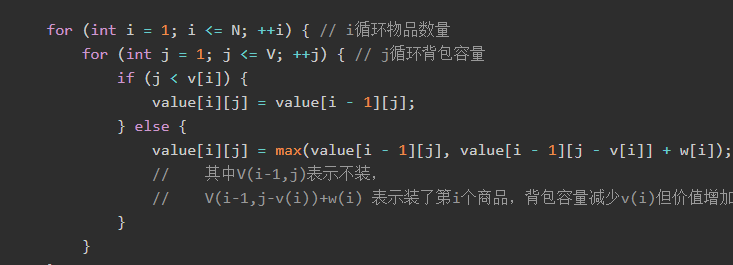# 01背包问题（二）

01背包的时间复杂度很难再降低了，但空间复杂度还能进行优化，可以把数组从二维降到一维。``````for (int i = 1; i <= N; ++i) { // i循环物品数量
for (int j = V; j >= v[i]; --j) { // j循环背包容量
// 这里要j >= v[i] 也就是背包容量j要大于该物品所需容量
if (j >= v[i])
// 这种题，一点小失误都会导致失败，这里要加=
value[j] = max(value[j], value[j - v[i]] + w[i]);
}
}``````

• 不放的话，和上式相同。
• 放的话，背包容量-第i件物品的质量再去装前i-1件物品，所得得最大价值加上第i件物品的价值，两者较大值即为最优解。d[j]=max(dp[j],dp[j-w[i]]+v[i])支付宝打赏微信打赏
]